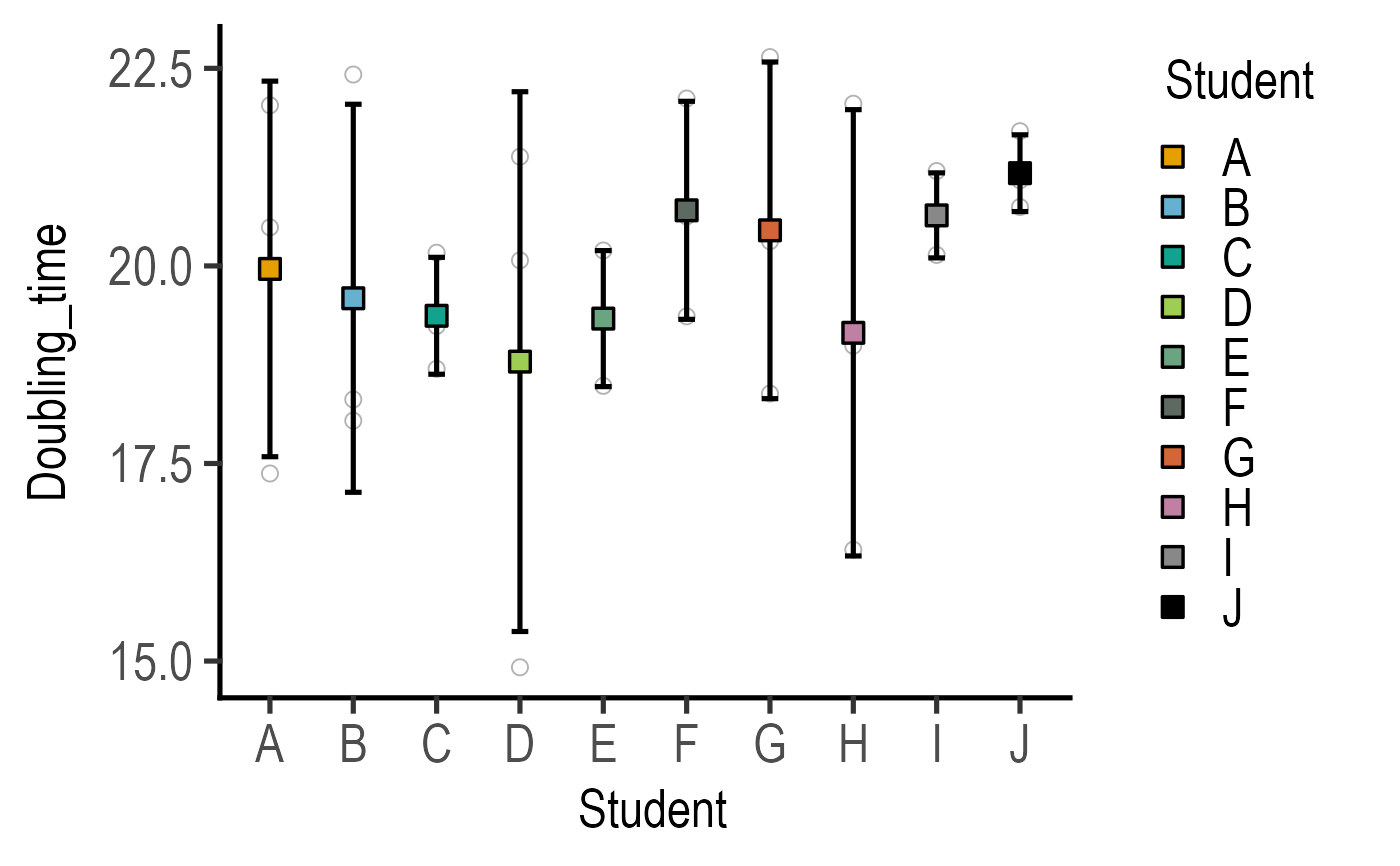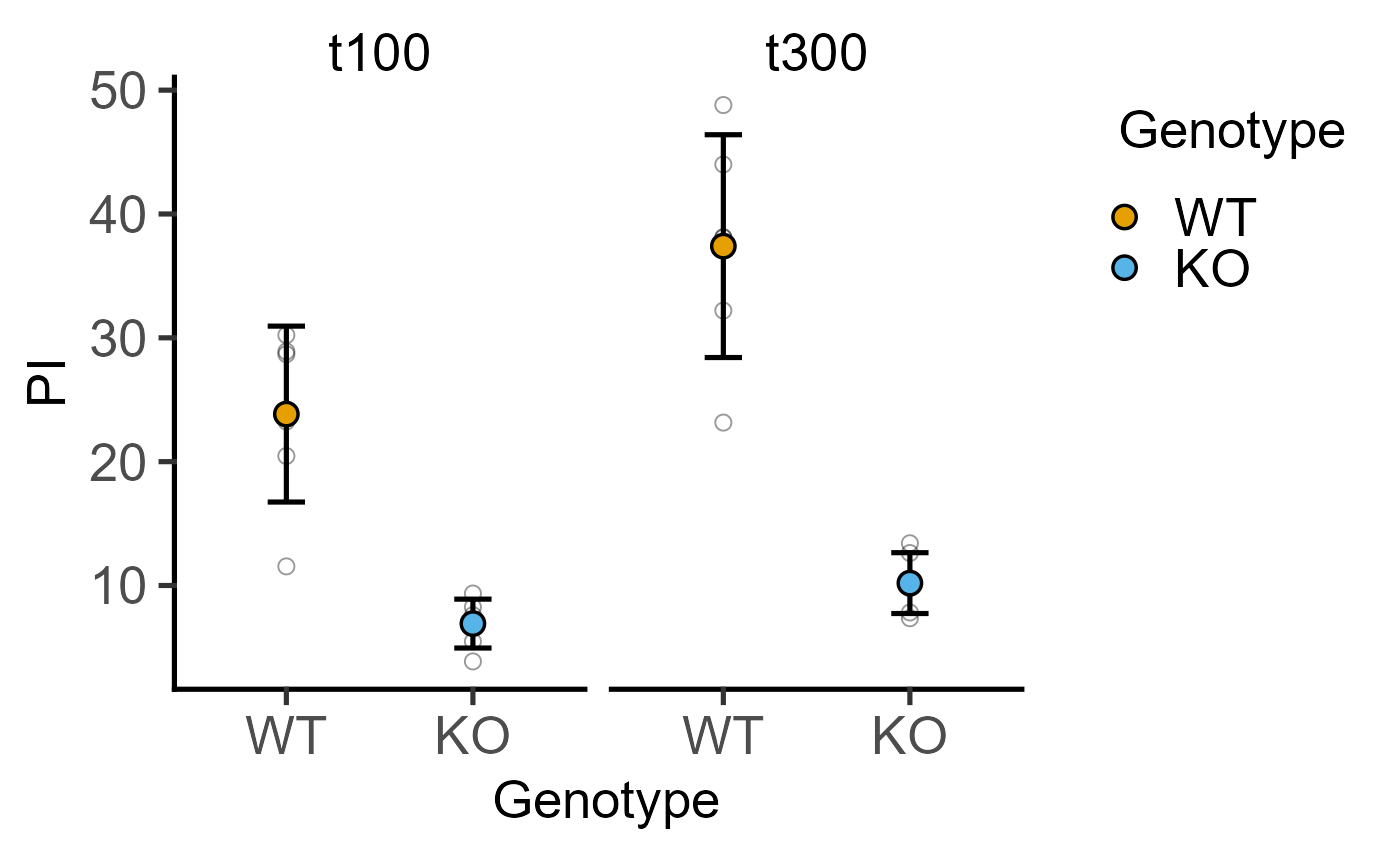This function takes a data table, categorical X and numeric Y variables, and plots a point showing the mean with SD error bars as default (SEM & CI95 are other options). The X variable is mapped to the fill aesthetic of symbols.

plot_point_sd(
data,
xcol,
ycol,
facet,
ErrorType = "SD",
symsize = 3.5,
s_alpha = 1,
symshape = 21,
all_alpha = 0,
all_size = 2.5,
all_shape = 1,
all_jitter = 0,
ewid = 0.2,
TextXAngle = 0,
LogYTrans,
LogYBreaks = waiver(),
LogYLabels = waiver(),
LogYLimits = NULL,
facet_scales = "fixed",
fontsize = 20,
symthick,
ethick,
ColPal = c("okabe_ito", "all_grafify", "bright", "contrast", "dark", "fishy", "kelly",
"light", "muted", "pale", "r4", "safe", "vibrant"),
ColSeq = TRUE,
ColRev = FALSE,
SingleColour = "NULL",
...
)

## Arguments

data

a data table object, e.g. data.frame or tibble.

xcol

name of the column with a categorical X variable.

ycol

name of the column with quantitative Y variable.

facet

add another variable from the data table to create faceted graphs using ggplot2facet_wrap.

ErrorType

select the type of error bars to display. Default is "SD" (standard deviation). Other options are "SEM" (standard error of the mean) and "CI95" (95% confidence interval based on t distributions).

symsize

size of point symbols, default set to 3.5.

s_alpha

fractional opacity of symbols, default set to 1 (i.e. maximum opacity & zero transparency).

symshape

The mean is shown with symbol of the shape number 21 (default, filled circle). Pick a number between 0-25 to pick a different type of symbol from ggplot2.

all_alpha

fractional opacity of all data points (default = 0; i.e., not shown). Set to non-zero value if you would like all data points plotted in addition to the mean.

all_size

size of symbols of all data points, if shown (default = 2.5).

all_shape

all data points are shown with symbols of the shape number 1 (default, transparent circle). Pick a number between 0-25 to pick a different type of symbol from ggplot2.

all_jitter

reduce overlap of all data points, if shown, by setting a value between 0-1 (default = 0).

ewid

width of error bars, default set to 0.2.

TextXAngle

orientation of text on X-axis; default 0 degrees. Change to 45 or 90 to remove overlapping text.

LogYTrans

transform Y axis into "log10" or "log2"

LogYBreaks

argument for ggplot2[scale_y_continuous] for Y axis breaks on log scales, default is waiver(), or provide a vector of desired breaks.

LogYLabels

argument for ggplot2[scale_y_continuous] for Y axis labels on log scales, default is waiver(), or provide a vector of desired labels.

LogYLimits

a vector of length two specifying the range (minimum and maximum) of the Y axis.

facet_scales

whether or not to fix scales on X & Y axes for all facet facet graphs. Can be fixed (default), free, free_y or free_x (for Y and X axis one at a time, respectively).

fontsize

parameter of base_size of fonts in theme_classic, default set to size 20.

symthick

thickness of symbol border, default set to fontsize/22.

ethick

thickness of error bar lines; default fontsize/22.

ColPal

grafify colour palette to apply, default "okabe_ito"; see graf_palettes for available palettes.

ColSeq

logical TRUE or FALSE. Default TRUE for sequential colours from chosen palette. Set to FALSE for distant colours, which will be applied using scale_fill_grafify2.

ColRev

whether to reverse order of colour within the selected palette, default F (FALSE); can be set to T (TRUE).

SingleColour

a colour hexcode (starting with #), a number between 1-154, or names of colours from grafify or base R palettes to fill along X-axis aesthetic. Accepts any colour other than "black"; use grey_lin11, which is almost black.

...

any additional arguments to pass to ggplot2stat_summary.

## Value

This function returns a ggplot2 object of class "gg" and "ggplot".

## Details

The function uses stat_summary with geom = "point" with size = 3. Standard deviation (SD) is plotted through stat_summary calculated using mean_sdl from the ggplot2 package (get help with ?mean_sdl), and 1x SD is plotted (fun.arg = list(mult = 1). mean_se and mean_cl_normal are used for SEM and CI95, respectively.

Colours can be changed using ColPal, ColRev or ColSeq arguments. Colours available can be seen quickly with plot_grafify_palette. ColPal can be one of the following: "okabe_ito", "dark", "light", "bright", "pale", "vibrant, "muted" or "contrast". ColRev (logical TRUE/FALSE) decides whether colours are chosen from first-to-last or last-to-first from within the chosen palette. ColSeq (logical TRUE/FALSE) decides whether colours are picked by respecting the order in the palette or the most distant ones using colorRampPalette.

If there are many groups along the X axis and you prefer a single colour for the graph,use the SingleColour argument.

You are instead encouraged to show all data using the following functions: plot_scatterbar_sd, plot_scatterbox, plot_dotbox, plot_dotbar_sd, plot_scatterviolin or plot_dotviolin.

## Examples

#Basic usage
plot_point_sd(data = data_doubling_time,
xcol = Student, ycol = Doubling_time)#show all data points
plot_point_sd(data = data_2w_Tdeath,
xcol = Genotype, ycol = PI,
facet = Time, all_alpha = 0.4)